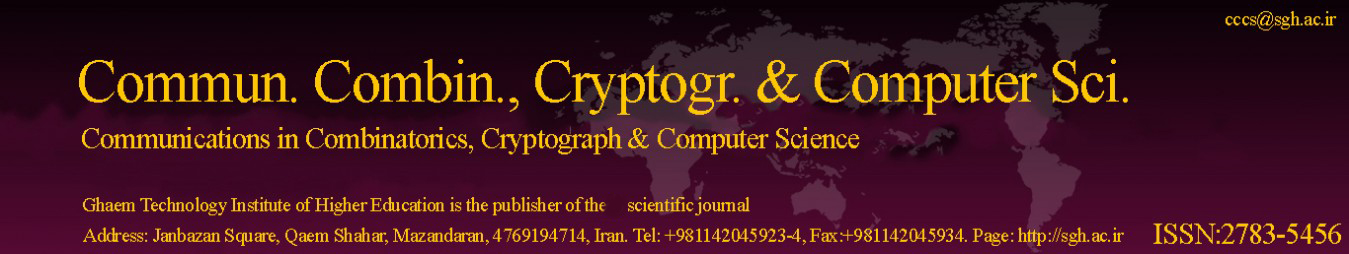Original Research Article

Article volume = 2021 and issue = 1

Pages: 89–91

Article publication Date: November, 1, 2021

# On The Tree-Number of Conjugacy Class Graphs of Some Metacyclic Groups

#### Zeinab Foruzanfar

Imam Khomeini International University - Buin Zahra Higher Education Center of Engineering and Technology, Qazvin, Iran.

##### Abstract:

For a finite group G with V(G) as the set of all non-central conjugacy classes of it, the conjugacy class graph 􀀀 (G) is defined as: its vertex set is the set V(G) and two distinct vertices aG and bG are connected with an edge if (o(a), o(b)) > 1. In this paper, we determine the tree-number of the conjugacy class graphs of metacyclic groups of order less than thirty.

##### Keywords:

Metacyclic group, conjugacy class graph, tree-number.

##### References:
•  R. B. Bapat, Graphs and Matrices, Springer, New York, (2010). 2.2
•  L. W. Beineke, R. J. Wilson, Topics in algebraic graph theory, 102, Cambridge University Press, New York, (2004). 2.1
•  N. Biggs, Algebraic Graph Theory, Cambridge University Press, Cambridge, Second edition, (1993). 1, 2.3
•  C. E. Hempel, Metacyclic Groups, Commun. Algebra, 28 (2000), 3865–3897. 1
•  J. S. Rose, A Course on Group Theory, New York: Dover, (1994). 1
•  X. You, G. Qian, A new graph related to conjugacy classes of finite groups, Chinese Ann. Math. Ser. A, 28(5) (2007), 631–636. 1